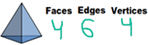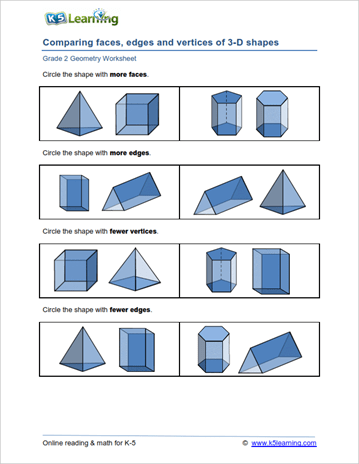# 2nd Grade Math Worksheets: Geometry

Our grade 2 geometry worksheets focus on the basic properties of two dimensional shapes as well as introducing the concepts of congruency, symmetry, area and perimeter. Our final worksheets introduce 3D shapes.

## Basic properties of 2-D shapes

Identifying 2-D Shapes
Circles, squares, rectangles, triangles, pentagons, hexagons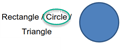Edges and vertices
Counting edges & vertices of common shapes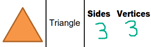## Composing and decomposing 2D shapes

Composing rectangles from squares
Creating rectangles by joining squares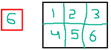Parts of a whole
Common shapes divided into equal parts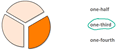## Congruency and symmetry of 2D shapes

Congruent shapes
Identify the congruent (same size, same shape) shapes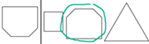Line of symmetry
Draw lines of symmetry, symmetrical shapes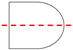## Area and perimeter of 2D shapes

Perimeters of 2D shapes
Common shapes, irregular rectangular shapes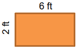Area of rectangles
As a sum of squares or a sum of triangles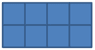## 3D Shapes

Identifying 3D shapes
Including matching shapes to real objects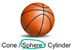Edges, faces and vertices
Properties of 3D shapes